# Kerala Syllabus 9th Standard Maths Solutions Chapter 8 Polynomials

## Kerala State Syllabus 9th Standard Maths Solutions Chapter 8 Polynomials

### Polynomials Textual Questions and Answers

Textbook Page No. 123

Polynomial Root Calculator solves the roots of polynomials easily.

Polynomials Class 9 Kerala Syllabus Chapter 8 Question 1.
In rectangles with one side 1 centimetre shorter than the other, take the length of the shorter side as x centimetres.
i. Taking their perimeters as p(x) centimetres, write the relation between p(x) and x as an equation,
ii. Taking their areas as a(x) square centimetres, write the relation between a(x) and x as an equation.
iii Calculate p(l), p(2), p(3), p(4), p(5). Do you see any pattern?
iv. Calculate a(l), a(2), a(3), a(4), a(5). Do you see any pattern?
Let x be the shorter side, then the other side will be (x+ 1).
i. Perimeter = 2[x + (x + 1)] = 2(2x + 1) = 4x + 2
That is, p(x) = 4x + 2

ii. Area = x(x + 1)
a(x) = x2 + x
Area, a(x) = x2 + x

iii. p(x) = 4x + 2
p(1) = 4 × 1 + 2 = 6
p(2) = 4 × 2 + 2 = 10
p(3) = 4 × 3 + 2= 14
p(4) = 4 × 4 + 2= 18
p(5) = 4 × 5 + 2 = 22
Perimeter is a sequence increasing by 4.

iv. a(x) = x2 + x
a(1) = 12 + 1 = 2 = 1 × 2 = 2
a(2) = 22 + 2 = 6 = 2 × 3 = 6
a(3) = 32 + 3 = 12 = 3 × 4 = 12
a(4) = 42 + 4 = 20 = 4 × 5 = 20
a(5) = 52 + 5 = 30 = 5 × 6 = 30
Area is the product of x and the number one more than x.

Polynomial Root Calculator solves the roots of polynomials easily.

Polynomials Class 9 State Syllabus Chapter 8 Question 2.
From the four corners of a rect-angle, small squares are cut off and the sides are folded up to make a box, as shown below: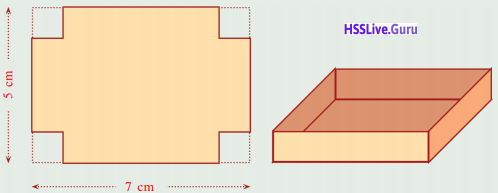i. Taking a side of the square as x centimetres, write the dimensions of the box in terms of x.
ii. Taking the volume of the box as vfojcubic centimetres, write the relation between v(x) and x as an equation.
iii. Calculate $$\mathrm{V}\left(\frac{1}{2}\right) \quad, \mathrm{V}(1), \quad \mathrm{V}\left(1 \frac{1}{2}\right)$$
If 1cm is the length of the small squares they are cut off, then the length of the maked box by folding it up = 7 – 1 – 1 = 5 cm Width = 5 – 1 – 1 = 3 cm, Height = 1 cm
If 2 cm is the length of the small squares that are cut off, then the length of the maked box by folding it up = 7 – 2 – 2 = 3 cm Width = 5 – 2 – 2 = 1 cm, Height = 2cm
i. If x cm is the length of the small squares that are cut off, then the length of the maked box by folding it up = 7 – x – x = (7 – 2x) cm
Width = 5 – x – x = (5 – 2x) cm
Height = x cm

ii. If volume of the box be v(x), then
v(x) = Length × Width × Height v(x) = (7 – 2x) (5 – 2x)x cm3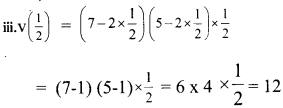v (1) = (7 – 2 × 1) (5 – 2 × 1) 1 = (7 – 2) (5 – 2) × 1 = 5 × 3 × 1 = 15Hss Live Guru 9th Maths Kerala Syllabus Chapter 8 Question 3.
Consider all rectangles that can be made with a 1-metre long rope. Take one of its sides as x centimetres and the area enclosed as a(x) square centimetres.
i. Write the relation between a(x) and x as an equation.
ii. Why are the numbers a(10) and a(40) equal?
iii. To get the same number as a(x), for two different numbers as x, what must be the relation between the numbers?
i. 1 m = 100 cm
If one side is x cm, then the other side is 50 – x.
Area of rectangle = a(x) = x(50 – x)
= 50x – x2 cm2
a(x) = 50x – x2

ii. a(10) = 50 × 10 – 102 = 500 – 100 = 400
a(40) = 50 × 40 – 402 = 2000 – 1600 = 400
10 and 40 are the sides of rectangle, so a(10) and a(40) are same .

iii. Numbers must be sides of rectangle. If we add the two numbers together we get the sum as half the length of the wire (50 cm) used to make it.

Textbook Page No. 126

Free Is Polynomial Calculator – Check whether a function is a polynomial step-by-step.

Hsslive Guru 9th Maths Kerala Syllabus Chapter 8 Question 1.
Write each of the relations below in algebra and see if it gives a polynomial. Also, give reasons for your conclusion.
i. A 1-metre wide path goes around a square ground. The relation between the length of a side of the ground and the area of the path.

ii A liquid contains 7 litres of water and 3 litres of acid. More acid is added to it. The relation between the amount of acid added and the change in the percentage of acid in the liquid.

iii. Two poles of heights 3 metres and 4metres are erected upright on the ground, 5 metres apart. A rope is to be stretched from the top of one pole to some point on the ground and from there to the top of the other pole: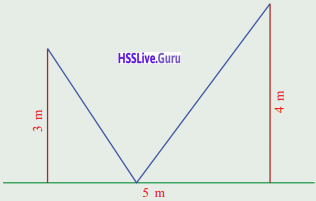The relation between the distance of the point on the ground from the foot of a pole and the total length of the rope.
i. Let the side of the ground be x then the side of the square including the path is x + 2 metres.
Area of path = Area of large square – Area of small square.
a(x) = (x + 2)2 – x2 = x2 + 4x +4 – x2
= 4x + 4
Here a(x) is a polynomial. Here x is multiplied by 4 and 4 is added to it.

ii. Ratio of acid in the first fluid = 3/10 litre that is 30%
If x litres of acid is added to it, then change in the amount of acid is
$$=\frac{3+x}{10+x}$$
Change in the percentage of acid is = $$\frac{3+x}{10+x} \times 100 \%$$
$$\mathrm{b}(\mathrm{x})=\frac{300+100 x}{10+x} \%$$

iii.In figure, AB = x m, BD = 5 – x
Total length of the wire = BC + BE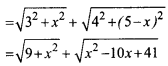It is not a polynomial since it involves square root of variable x.

Sum and difference of cubes calculator, multiplying and dividing rational exponents not possible.

Kerala Syllabus 9th Standard Maths Notes Chapter 8 Question 2.
Write each of the operations below as an algebraic expression, find out which are polynomials and explain why.
i. Sum of number and it’s reciprocal
ii. Sum of a number and its square root.
iii. Product of the sum and difference of a number and its square root.
i. Let the number be x, then the reciprocal is 1/x
sum = $$x+\frac{1}{x}$$
This is not a polynomial, because here the operation of reciprocal is involved.

ii. Let the number be x, then the square root is √x
sum = x + √x
This not a polynomial because here the square root is taken.

iii. Let x be the number
(x + √x) (x – √x) = x2– x
This is a polynomial.

Std 9 Maths Notes Kerala Syllabus Chapter 8 Question 3.
Find p(1) and p(10) in the following polynomials,
i. p(x) = 2x + 5
ii. p(x) = 3x2 + 6x + 1
iii. p(x) = 4x3 + 2x2 + 3x + 7
i. p(x) = 2x + 5
p(1) = 2 × 1 + 5 = 2 + 5 = 7
p(10) = 2 × 10 + 5 = 20 + 5 = 25

ii. p(x) = 3x2 + 6x + 1
p(1) = 3 × 12 + 6 x 1 + 1
= 3 + 6 + 1 = 10
p(10) = 3 × 102 + 6 × 10 + 1 = 300 + 60 + 1 = 361

iii. p(x) = 4x3 + 2x2 + 3x + 7
p(1) = 4 × 13 + 2 × 12 + 3 × 1 +7
=4 + 2 + 3 + 7 = 16
p(10) = 4 × 103 + 2 × 102 + 3 × 10 + 7
= 4000 + 200 + 30 + 7 = 4237

Hss Live Maths 9th Kerala Syllabus Chapter 8 Question 4.
Find p(0), p(1) and p(-1) in the fol-lowing polynomials,
i. p(x) = 3x + 5
ii. p(x) = 3x2 + 6x + 1
iii. p(x) = 2x2 – 3x + 4
iv. p(x) = 4x3 + 2×2 + 3x + 7
v. p(x) = 5x3 – x2 + 2x – 3
i. p(x) = 3x + 5
p(0) = 3 × 0 + 5 = 5
p(1) = 3 × 1 + 5 = 8
p(-1) = 3 x-1 + 5 = 2

ii. p(x) = 3x2 + 6x + 1
p(0) = 3 × 02 + 6 × 0 + 1 = 1
p(1) = 3 × 12 + 6 × 1 + 1 = 10
p(-1) = 3 × (-1)2 + 6 × (-1) + 1 = -2

iii. p(x) = 2x2 – 3x + 4
p(0) = 2 × 02 – 3 × 0 + 4 = 4
p(1) = 2 × 12 – 3 × 1 + 4 = 3
p(-1) =2 × (-1)2 – 3 × (-1) + 4 = 9

iv. p(x) = 4x3 + 2x2 + 3x + 7
p(0) = 4 × 03 + 2 × 02 + 3 × 0 +7 = 7
p(1) = 4 × 13 + 2 × 12 + 3 × 1 + 7 = 16
p(-1) = 4 × (-1)3 + 2 × (-1)2 + 3 × (-1) +7 =2

v. p(x) = 5x3 – x2 + 2x – 3
p(0) = 5 × 03 – 02 + 2 × 0 – 3 =-3
p(1) = 5 × 13 – 12 + 2 × 1 – 3 = 3
p(-1) = 5 × (-1)3 – (-1)2 + 2 × (-1) – 3 = -11

Scert Class 9 Maths Solutions Kerala Syllabus Chapter 8 Question 5.
Find polynomials p(x) satisfying
each set of conditions below.
i. First degree polynomials with p(1) = 1 and p(2) = 3
ii. First degree polynomials with p(1) = -1 and p(-2) = 3
iii. Second degree polynomials with p(0) = 0, p(1) = 2 and p(2) = 6.
iv. Three different second degree polynomials with p(0) = 0 and p(1) = 2.
i. General form of a first degree poly-nomial is
p(x) = ax + b
Let p(1) = 1, then a × 1 + b = l
a + b = 1 ………. (1)
Let p(2) = 3, then a × 2 + b = 3
2a + b = 3 …….. (2)
(1) × 2, 2a + 2b = 2 …… (3)
(3) – (2), b = -1
From (1), a + -1 = 1,
a = 1 + 1 = 2 Polynomial p(x) = 2x – 1

ii. General form of a first degree poly-nomial is p(x) = ax + b
Let p(1) = -1, then a × 1 + b = -l
a + b = -1 ……….. (1)
Let p(-2) =3 , then a × (-2) + b = 3
-2a + b = 3 ………. (2)
(1) × 2, 2a + 2b = -2 ………. (3)
(2) + (3), 3b = 1, b = 1/3
From (1), a = 1/3 = -1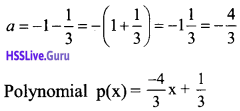iii. General form of a second degree polynomial is
p(x) = ax2 + bx + c
Letp(0) = 0, then a × 02 + b × 0 + c = 0
0a + 0b + c = 0.
c = 0 (1)
Letp(1) = 2 ,then a × 12 + b × 1 + c = 2
a + b + 0 = 2
a + b = 2 ………. (2)
Letp(2)= 6, then a × 22 + b × 2 + c = 6
4a + 2b = 6
2a + b = 3 (3)
(3) – (2), a = 1
From (2), 1 + b = 2, b = 2 – 1 = 1
Polynomial p(x) = x2 + x

iv. General form of a second degree polynomial is p(x) = ax2 + bx + c
Letp(0) = 0, then a x 0 + b x 0 + c = 0
0 + 0 + c = 0
c = 0
Letp(1) = 2, then a × 12 + b × 1 + c = 2
a + b + c = 2
a + b = 2
Selecting a and b such that a + b = 2 will give different polynomials.
a = 1, b = 1
a= 3, b =-l
a=4, b =-2
Three different second degree poly¬nomials are
p(x) = x2 + x
p(x) = 3x2 – x
p(x) = 4x2 – 2x

### Polynomials Exam Oriented Questions and Answers

Hss Live 9th Maths Kerala Syllabus Chapter 8 Question 1.
In a polynomial p(x) = 2x3 + ax2 – 7x + b.
p(1) = 3, p(2) = 19. Then find the value of ‘a’ and ‘b’?
p(x) = 2x3 + ax2 – 7x + b
We have p(1) = 3.
p(1) = 2 x 13 + a x 12 – 7 x 1 + b = 3
2 + a – 7 + b = 3
a + b – 5 = 3
a + b =8 ………. (1)
We have p(2) = 19.

p(2 ) = 2 x 23 + a x 22– 7 x 2 + b = 19
16 + 4a – 14 + b = 19
4a + b + 2 = 19
4a + b = 17…….. (2)
From equation (1), (2)
(2) – (1) 4a + b = 17
$$\frac{a+b=8}{3 a=9}$$
a = 9/3 = 3
From equation (1)
a + b = 8
3+b =8
b =5

Hsslive Class 9 Maths Kerala Syllabus Chapter 8 Question 2.
In a polynomial p(x) = 2x3 + 9x2 + kx + 3, p(-2) = p(-3). Find the value of k.
p(x) = 2x3 + 9x2 + kx + 3
p(-2) = 2(-2)3 + 9(-2)2 + k(-2) + 3
= -16 + 36 – 2k + 3 = 23 – 2k
p(-3) = 2(-3)3 + 9(-3)2 + k(-3) + 3
= -54 + 81 – 3k + 3 = 30 – 3k
We have p(-2) = p(-3),
23 -2k = 30 -3k
-2k + 3k = 30 – 23
k = 7

Maths Kerala Syllabus Std 9 Notes Chapter 8 Question 3.
From the polynomial p(x) = 2x – 3x +1, find p(0), p(1) and p(-1).
p(x) = 2x2 – 3x +1
p(0) = 2(0)2 – 3(0) + 1 = 1
p(1) = 2(1)2 – 3(1) +1 = 2 – 3 + 1 = 0
p(-1) = 2(-1)2 – 3(-1) + 1 = 2 + 3 + 1 = 6

Kerala Syllabus 9th Standard Maths Notes Pdf Chapter 8 Question 4.
In a polynomial p(x) = 2x3 – 7x2 + kx + 20,
p(2) = p(3).
a. Find the value of k.
b. Using the value of k, write the polynomial.
c. Find p(1).
a. p(2) = 2 × 22 – 7 × 22 + k × 2 + 20
= 16 – 28 + 2k + 20 = 8+ 2k
p(3) = 2 × 33 – 7 × 32+ k × 3 + 20
= 54 – 63 + 3k + 20 = 11 +3k
P(2) = P(3)
8 + 2k = 11 + 3k
k = -3

b. p(x) = 2x3 – 7x2 -3x + 20

c. p(1) = 2 – 7 – 3 + 20 = 12

Chapter 8 Polynomials Answers Kerala Syllabus Question 5.
Simplify the followi ng
i. (2x + 1) (3x + 4) (4x + 3) (3x + 4)
ii. (3x + 4)2 – (2x – 1) (3x + 4)
i. (2x + 1) (3x + 4) (4x + 3) (3x + 4)
= (3x + 4) [(2x + 1 + 4x + 3)]
= (3x + 4)[6x + 4]
= (3x x (6x) + (3x) x (4)+ 4x(6x) + 4 x 4
= 18x2 + 12x + 24x + 16
= 18x2 + 36x + 16

ii. (3x + 4)2 – (2x – 1) (3x + 4)
= (3x + 4) [3x + 4 – (2x – 1)]
= (3x + 4)[3x + 4 – 2x + 1] = (3x + 4) (x + 5)
= 3x2 + 15x + 4x + 20
= 3x2 + 19x + 20

Hsslive Guru Maths 9th Kerala Syllabus Chapter 8 Question 6.
7x3 – 4x2 – x + 4 is a polynomial.
a. Write the terms of the polynomial.
b. Write the coefficient of x2.
c. Write the constant terms of the polynomial.
d. What is the degree of the polynomial ?
a. Terms = 7x2, -4x2, -x, 4
b. Coefficient of x2 = -4
c. Constant term = 4
d. Degree of the polynomial = 3

Hss Live Guru Class 9 Maths Kerala Syllabus Chapter 8 Question 7.
In the polynomial p(x)=3x2 – ax + 1,
Find ‘a’ satisfying p(1) = 2.
p(x)=3x2 – ax + 1
p(1) = 3(1)2 – (a × 1) + 1 = 3 – a + 1 =4 – a
Given p(1) = 2
That is, 4 – a = 2 , a = 4 – 2 = 2

Hsslive Maths Class 9 Kerala Syllabus Chapter 8 Question 8.
If p(x)= x3 + 2x2 – 3x + 1 and q(x) = x3 – 2x2 + 3x + 5
a) Find p(x) + q(x). What is its de-gree?
b) Find p(x) – q(x). What is its de-gree?
a) p(x) = x3 + 2x2 – 3x + 1,
q(x) = x3 – 2x2 + 3x + 5
p(x) + q(x)
= x3 + 2x2 – 3x + 1 + x3 – 2x2 + 3x + 5
= 2x3 + 6
Degree = 3

b) p(x) – q(x)
= x3 + 2x2 – 3x + 1 – (x3 – 2x2 + 3x + 5)
= x3 + 2x2 – 3x + 1 -x3 + 2x2 – 3x – 5
= 4x2 – 6x – 4
Degree = 2

9th Std Maths Notes Kerala Syllabus Chapter 8 Question 9.
A right-angled triangle of perpendicular sides 3 cm and 4 cm are ex-tended equally then get another large right-angled triangle. Write the algebraic form of the hypotenuse of the large right-angled triangle.
Hypotenuse 2 = base 2 + height 2
(According to pythagorus theorem)
Let the perpendicular side be x cm Length of other sides = 3 + x cm, 4 + x cmQuestion 10.
In the polynomial p(x)= 3x2 – 4x + 7, check whether p(1) + p(2) = p(3) and P(2) × p(3) = p(6).
p(x) = 3x2 – 4x + 7
p(1) = 3(1)2 – 4(1) + 7 = 3 – 4 + 7 = 6
p(2) = 3(2)2 -4(2) + 7 = 12 – 8 + 7 = 11
p(3) = 3(3)2 – 4(3) +7 = 27 – 12 + 7 = 22
p(l) + p(2) ≠ p(3)
p(6) = 3(6)2 -4(6) + 7 = 108 – 24 + 7 = 91
P(2) × p(3) ≠ p(6)

Question 11.
In the polynomial p(x)= 2x2 + ax2 – 7x + b,
p(1) = 3 and p(2) = 19. Find a and b.
p(x)=2x2 + ax2 – 7x + b
p(l)=2 x 13+ a x 12 – 7 x 1 +b
=2 + a – 7 + b =a + b + 2 – 7 = a + b – 5
p(1) = 3, a + b – 5 = 3
a + b = 8……… (1)
p(2) = 2 x 23 + a x 22 – 7 x 2 + b
= 16 + 4a – 14 + b = 4a + b + 2
p(x) = 19
4a + b + 2 = 19
4a + b = 17 ……….. (2)
(2) – (1)
3a = 9, a = 3 a + b – 5 = 3
From equation (1),
a + b = 8
3 + b = 8
b = 5

Question 12.
If p(x) = x2 + 3x + 1 and q(x) = 2x – 4, then
i. Find the degree of the polyno-mial p(x) q(x).
ii. If the degree of p(x) × r(x) is 5, then find the degree of the polynomial r(x) .
iii. If p(x) is a third degree poly-nomial and q(x) is a fourth de-gree polynomial then find the degree of p(x) × q(x).
i. p(x) = x2 + 3x + 1 , q(x) = 2x – 4
p(x) × q(x) = (x2 + 3x + 1) (2x – 4)
The degree of p(x) x q(x) is 3.

ii. p(x) = x2 + 3x + 1 is a second degree polynomial.
If the degree of the polynomial p(x) × r(x) is 5, then p(x) × r(x) must have a term of x5.
x2 × x3 = x5
So, r(x) is a third-degree polynomial.

iii. If p(x) is a third-degree polynomial, then p(x) must have a term of x3.
q(x) is a fourth-degree polynomial, then q(x) must have a term of x4
Then in p(x) × q(x), must have a term of x3 × x4= x7
So p(x) × q(x) is a seventh-degree polynomial. In general,
If p(x) is an mth degree polynomial and q(x) is an n,h degree polynomial then p(x) × q(x) is an (m + n),h degree polynomial.

Question 13.
If p(x) = 4x2 + 3x + 5 and q(x) = 3x2 – x – 7, then find p(x) + q(x) and p(x) – q(x).
p(x) + q(x) = (4x2 + 3x + 5) + (3x2 – x – 7)
= 4x2 + 3x2 + 3x – x + 5 – 7
= 7x2 + 2x – 2
p(x) – q(x) = (4x2 + 3x + 5) – (3x2 – x – 7)
= 4x2 + 3x + 5 – 3x2 + x + 7
= x2 + 4x + 12

Question 14.
In polynomial p(x) = ax3+ bx2 + cx + d, p(l) = p(-l). Prove that a + c = 0.
p(x) = ax3 + bx2 + cx + d
p(l) = a(1)3 + b(1)2 + c(1) + d
= a+ b + c + d
p(-1) = a(-1)3 + b(-1)2 +c(-1) + d
= -a + b – c + d
p(1) = p(-1) is given ie, a + b + c + d = -a + b – c + d
2a + 2c = 0; 2(a + c ) = 0;
ie, a + c = 0

Question 15.
If we divide a polynomial by (x-2) we get quotient as x2 + 1 and remainder as 5. Find the polynomial.